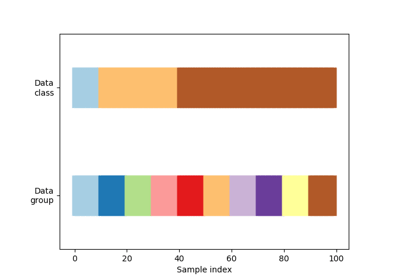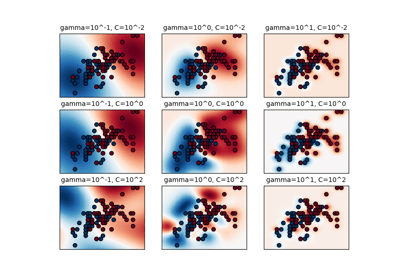/scikit-learn

# sklearn.model_selection.StratifiedShuffleSplit

`class sklearn.model_selection.StratifiedShuffleSplit(n_splits=10, test_size=’default’, train_size=None, random_state=None)` [source]

Stratified ShuffleSplit cross-validator

Provides train/test indices to split data in train/test sets.

This cross-validation object is a merge of StratifiedKFold and ShuffleSplit, which returns stratified randomized folds. The folds are made by preserving the percentage of samples for each class.

Note: like the ShuffleSplit strategy, stratified random splits do not guarantee that all folds will be different, although this is still very likely for sizeable datasets.

Read more in the User Guide.

Parameters: `n_splits : int, default 10` Number of re-shuffling & splitting iterations. `test_size : float, int, None, optional` If float, should be between 0.0 and 1.0 and represent the proportion of the dataset to include in the test split. If int, represents the absolute number of test samples. If None, the value is set to the complement of the train size. By default, the value is set to 0.1. The default will change in version 0.21. It will remain 0.1 only if `train_size` is unspecified, otherwise it will complement the specified `train_size`. `train_size : float, int, or None, default is None` If float, should be between 0.0 and 1.0 and represent the proportion of the dataset to include in the train split. If int, represents the absolute number of train samples. If None, the value is automatically set to the complement of the test size. `random_state : int, RandomState instance or None, optional (default=None)` If int, random_state is the seed used by the random number generator; If RandomState instance, random_state is the random number generator; If None, the random number generator is the RandomState instance used by `np.random`.

#### Examples

```>>> from sklearn.model_selection import StratifiedShuffleSplit
>>> X = np.array([[1, 2], [3, 4], [1, 2], [3, 4], [1, 2], [3, 4]])
>>> y = np.array([0, 0, 0, 1, 1, 1])
>>> sss = StratifiedShuffleSplit(n_splits=5, test_size=0.5, random_state=0)
>>> sss.get_n_splits(X, y)
5
>>> print(sss)
StratifiedShuffleSplit(n_splits=5, random_state=0, ...)
>>> for train_index, test_index in sss.split(X, y):
...    print("TRAIN:", train_index, "TEST:", test_index)
...    X_train, X_test = X[train_index], X[test_index]
...    y_train, y_test = y[train_index], y[test_index]
TRAIN: [5 2 3] TEST: [4 1 0]
TRAIN: [5 1 4] TEST: [0 2 3]
TRAIN: [5 0 2] TEST: [4 3 1]
TRAIN: [4 1 0] TEST: [2 3 5]
TRAIN: [0 5 1] TEST: [3 4 2]
```

#### Methods

 `get_n_splits`([X, y, groups]) Returns the number of splitting iterations in the cross-validator `split`(X, y[, groups]) Generate indices to split data into training and test set.
`__init__(n_splits=10, test_size=’default’, train_size=None, random_state=None)` [source]
`get_n_splits(X=None, y=None, groups=None)` [source]

Returns the number of splitting iterations in the cross-validator

Parameters: `X : object` Always ignored, exists for compatibility. `y : object` Always ignored, exists for compatibility. `groups : object` Always ignored, exists for compatibility. `n_splits : int` Returns the number of splitting iterations in the cross-validator.
`split(X, y, groups=None)` [source]

Generate indices to split data into training and test set.

Parameters: `X : array-like, shape (n_samples, n_features)` Training data, where n_samples is the number of samples and n_features is the number of features. Note that providing `y` is sufficient to generate the splits and hence `np.zeros(n_samples)` may be used as a placeholder for `X` instead of actual training data. `y : array-like, shape (n_samples,)` The target variable for supervised learning problems. Stratification is done based on the y labels. `groups : object` Always ignored, exists for compatibility. `train : ndarray` The training set indices for that split. `test : ndarray` The testing set indices for that split.

#### Notes

Randomized CV splitters may return different results for each call of split. You can make the results identical by setting `random_state` to an integer.

## Examples using `sklearn.model_selection.StratifiedShuffleSplit`Visualizing cross-validation behavior in scikit-learnRBF SVM parameters

© 2007–2018 The scikit-learn developers